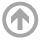Teaching# SM121 Calculus 1

On this page, you will find information for SM121, Calculus 1. Most resources will be posted to Google Classroom.

Course Objectives: Upon successful completion of this course, students will be able to:

1. Interpret, analyze, create, and communicate mathematical models involving derivatives.
2. Carry out computations involving order of growth, limits and derivatives.
3. Describe relations between geometry, formulas, and data.
4. Recognize and apply mathematical procedures to solve applied problems, including related rates and optimization.
5. Write simple proofs of mathematical results.

Textbook: "CALCULUS, Early Transcendentals", Eighth Edition, by James Stewart